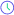Vidyakul : Delivering Education to Society11th Physics: Ch. 3: Motion In A Straight Line

Physics Hinglish Total Chapters: 1
Arvind Sir

Motion in a Straight Line

• Introduction
• Position, path length and displacement
• Average Velocity and Average Speed
• Instantaneous Velocity and Speed
• Acceleration
• Kinematic equations for uniformly accelerated motion
• Relative Velocity

Motion In A Straight Line

•3.1 Introduction , Understanding Reference Point43 Minutes
•3.2 Distance, Displacement, Velocity, Speed Etc.38 Minutes
•3.3 Understanding Acceleration And Uniform Acceleration34 Minutes
•3.4 Kinematics Equations Derivations15 Minutes
•3.5 Integration For Physics, Mathematical Tool16 Minutes
•3.6 Kinematics Equations Proof By Calculus Method23 Minutes
•3.7 Motion Under Gravity38 Minutes
•3.8 Kinematics Equations Proof By Graphical Method24 Minutes
•3.9 Kinematics, Understanding Graphs34 Minutes
•3.10 Numericals On Graph20 Minutes
•3.11 Relative Motion And Relative Velocity52 Minutes

Full Live Courses

See All
Physics Hindi24 Jan 09:00 AM

Vidyakul
Physics Hindi24 Jan 07:00 PM

Dr. Sushil Kukreti
Chemistry Hindi24 Jan 09:00 AM

Vidyakul
Physics Hinglish24 Jan 04:00 PM

Vidyakul